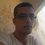A floor integral

Evaluate : $\int\limits_0^1\left\lfloor\frac{2}{x}\right\rfloor-2\left\lfloor\frac{1}{x}\right\rfloor\ \mathrm{d}x.$Note by Haroun Meghaichi
5 years, 3 months ago

This discussion board is a place to discuss our Daily Challenges and the math and science related to those challenges. Explanations are more than just a solution — they should explain the steps and thinking strategies that you used to obtain the solution. Comments should further the discussion of math and science.

When posting on Brilliant:

• Use the emojis to react to an explanation, whether you're congratulating a job well done , or just really confused .
• Ask specific questions about the challenge or the steps in somebody's explanation. Well-posed questions can add a lot to the discussion, but posting "I don't understand!" doesn't help anyone.
• Try to contribute something new to the discussion, whether it is an extension, generalization or other idea related to the challenge.
• Stay on topic — we're all here to learn more about math and science, not to hear about your favorite get-rich-quick scheme or current world events.

MarkdownAppears as
*italics* or _italics_ italics
**bold** or __bold__ bold

- bulleted
- list

• bulleted
• list

1. numbered
2. list

1. numbered
2. list
Note: you must add a full line of space before and after lists for them to show up correctly
paragraph 1

paragraph 2

paragraph 1

paragraph 2

> This is a quote
This is a quote
# I indented these lines
# 4 spaces, and now they show
# up as a code block.

print "hello world"
# I indented these lines
# 4 spaces, and now they show
# up as a code block.

print "hello world"
MathAppears as
Remember to wrap math in $$...$$ or $...$ to ensure proper formatting.
2 \times 3 $2 \times 3$
2^{34} $2^{34}$
a_{i-1} $a_{i-1}$
\frac{2}{3} $\frac{2}{3}$
\sqrt{2} $\sqrt{2}$
\sum_{i=1}^3 $\sum_{i=1}^3$
\sin \theta $\sin \theta$
\boxed{123} $\boxed{123}$

Sort by:

C

- 5 years, 2 months ago

Looking at the rectangular areas under the line segments given by the two floor functions:

$\int_0^1 \lfloor \frac{2}{x} \rfloor \mathrm{d}x \\ = 2\left(\frac{2}{2}-\frac{2}{3}\right)+3\left(\frac{2}{3}-\frac{2}{4}\right)+4\left(\frac{2}{4}-\frac{2}{5}\right)+5\left(\frac{2}{5}-\frac{2}{6}\right)+... \\ = \sum_{n=2}^{\infty} n\left(\frac{2}{n}-\frac{2}{n+1}\right) \\ = 2 \sum_{n=2}^{\infty} \frac{1}{n+1} \\ \int_0^1 \lfloor \frac{1}{x} \rfloor \mathrm{d}x \\ = 1\left(\frac{1}{1}-\frac{1}{2}\right)+2\left(\frac{1}{2}-\frac{1}{3}\right)+3\left(\frac{1}{3}-\frac{1}{4}\right)+4\left(\frac{1}{4}-\frac{1}{5}\right) ... \\ = \sum_{n=1}^{\infty} n \left( \frac{1}{n}-\frac{1}{n+1} \right) \\ = \sum_{n=1}^{\infty} \frac{1}{n+1} 2 \sum_{n=2}^{\infty} \frac{1}{n+1} - 2 \sum_{n=1}^{\infty} \frac{1}{n+1} = -2 \left( \frac{1}{2} \right) = -1$

- 5 years, 3 months ago

2*log(2) -1

- 5 years, 3 months ago

I'm a little confused. First I split the integral into two

$\int_{0}^{1} \left \lfloor \frac{2}{x} \right \rfloor dx - 2\int_{0}^{1} \left \lfloor \frac{1}{x} \right \rfloor dx$

In the first one I substituted x = 2t, to get

$2\int_{0}^{1/2} \left \lfloor \frac{1}{t} \right \rfloor dt - 2\int_{0}^{1} \left \lfloor \frac{1}{x} \right \rfloor dx$

which equals

$-2 \int_{1/2}^{1} \left \lfloor \frac{1}{t} \right \rfloor dt$

Now since t lies between 1/2 and 1, 1/t will lie between 1 and 2, therefore the integral will change to

$-2 \int_{1/2}^{1} 1 dt$

which will evaluate to -1

- 5 years, 3 months ago

With the substitution $\dfrac{1}{x}=t$, the integral is:

$\displaystyle \int_1^{\infty} \frac{\lfloor 2t \rfloor-2\lfloor t \rfloor}{t^2}\,dt = \sum_{n=1}^{\infty} \left(\int_n^{n+1/2} \frac{(2n)-2(n)}{t^2}\,dt+\int_{n+1/2}^{n+1} \frac{(2n+1)-2(n)}{t^2}\,dt \right)$

$\displaystyle =\sum_{n=1}^{\infty} \int_{n+1/2}^{n+1} \frac{(2n+1)-2(n)}{t^2}\,dt =\sum_{n=1}^{\infty} \left(\frac{2}{2n+1}-\dfrac{2}{2n+2}\right)$

$\displaystyle =2\left(\frac{1}{3}-\frac{1}{4}+\frac{1}{5}-\frac{1}{6}+\cdots \right)=2\left(\left(1-\frac{1}{2}+\frac{1}{3}-\frac{1}{4}+\cdots \right)-\frac{1}{2}\right)=\boxed{2\ln 2-1}$

- 5 years, 3 months ago

I'm a little confused. First I split the integral into two

$\int_{0}^{1} \left \lfloor \frac{2}{x} \right \rfloor dx - 2\int_{0}^{1} \left \lfloor \frac{1}{x} \right \rfloor dx$

In the first one I substituted x = 2t, to get

$2\int_{0}^{1/2} \left \lfloor \frac{1}{t} \right \rfloor dt - 2\int_{0}^{1} \left \lfloor \frac{1}{x} \right \rfloor dx$

which equals

$-2 \int_{1/2}^{1} \left \lfloor \frac{1}{t} \right \rfloor dt$

Now since t lies between 1/2 and 1, 1/t will lie between 1 and 2, therefore the integral will change to

$-2 \int_{1/2}^{1} 1 dt$

which will evaluate to -1

- 5 years, 3 months ago

Exactly my method to do it. Good job. ^ There is a small remark, if $n$ is an integer we may not always get : $\int_a^{\infty} f(t)\ \mathrm{d}t = \lim_{n\to \infty} \int_a^n f(t) \ \mathrm{d}t$ For example $f(t)=\sin(2\pi t)$, the first step should be checking whether it converges, if it does we can use this trick.

- 5 years, 3 months ago

My approach went like this:

First, note that

$\displaystyle \int_0^1 \left\lfloor\dfrac{1}{x}\right\rfloor\;dx = \displaystyle\lim_{N\to\infty} \int_{1/N}^1 \left\lfloor\dfrac{1}{x}\right\rfloor\;dx$

$\left\lfloor\dfrac{1}{x}\right\rfloor = n$ whenever $n\leq \dfrac{1}{x} < n+1$, or $\dfrac{1}{n+1}. This means that

$\displaystyle \int_{1/(n+1)}^{1/n} \left\lfloor\dfrac{1}{x}\right\rfloor\;dx = \displaystyle \int_{1/(n+1)}^{1/n} n\;dx = n\left(\dfrac{1}{n}-\dfrac{1}{n+1}\right)$ for every $n\in\mathbb{N}$

This now leads us to split the floor integral into a sum, with intervals $(1/(n+1),1/n]$:

$\displaystyle\lim_{N\to\infty} \int_{1/N}^1 \left\lfloor\dfrac{1}{x}\right\rfloor\;dx =\displaystyle\lim_{N\to\infty} \sum_{n=1}^{N-1} \displaystyle \int_{1/(n+1)}^{1/n} n\;dx$.

Working in a similar way with $\left\lfloor\dfrac{2}{x}\right\rfloor$, we find that our integral is supposed to be equal to

$\displaystyle\lim_{N\to\infty}\left( \sum_{m=2}^{N-1} \displaystyle \int_{2/(m+1)}^{2/m} m\;dx-2\sum_{n=1}^{N-1} \displaystyle \int_{1/(n+1)}^{1/n} n\;dx \right)$.

After making the lower limit of the sums equal, the variables similar and expanding the integrals, the sums should cancel out and what is left is one term

$-2\displaystyle \int_{1/(n+1)}^{1/n} n\;dx$ when $n=1$

as a result of making the lower limits equal. This is equal to $\boxed{-1}$

- 5 years, 3 months ago

The upper bound in the $m$ sum should be $2N-1$.

- 5 years, 3 months ago

thanks haha, ok let me rewrite. I was thinking, "wait this is answer is too nice"

- 5 years, 3 months ago

The exact answer is $\log(4)-1=0.386294...$, which is from the infinite sum

$\displaystyle\sum _{ n=1 }^{ \infty }{ \left(\dfrac { 2 }{ 2n+1 } -\dfrac { 2 }{ 2n+2 } \right) }$

Edited your $\LaTeX$

- 5 years, 3 months ago